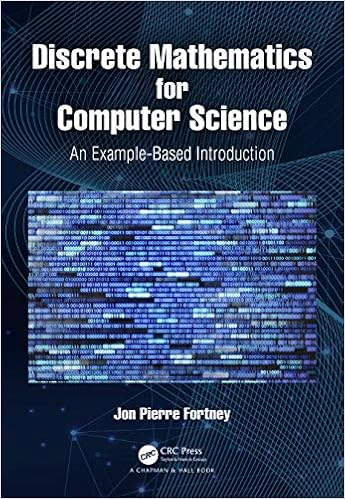Fortney, Jon Pierre
Discrete mathematics for computer science : an example-based introduction
Boca Raton, Florida : CRC Press, 2021. xii, 257 p.
QA76.9M35 F68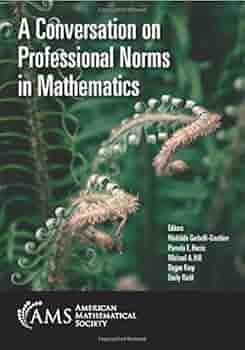Gerbelli-Gauthier, Mathilde (Ed.)
A conversation on professional norms in mathematics
Providence, Rhode Island : American Mathematical Society, 2021. x, 138 p.
QA12 C665Al-Baali, Mehidden (Ed.)
Numerical analysis and optimization
Cham, Switzerland : Springer, 2018. xvi, 304 p. -- (Springer proceedings in mathematics & statistics ; vol. 235)
QA297 I574 2017Ali, Akbar
Irregularity in graphs
Cham, Switzerland : Springer, 2021. x, 109 p. -- (SpringerBriefs in mathematics)
QA166 A5435Alpay, Daniel
Quaternionic de Branges spaces and characteristic operator function
Cham, Switzerland : Springer, 2020. x, 116 p. -- (SpringerBriefs in mathematics)
QA196 A46Jones, Gareth A.
Isomorphisms, symmetry and computations in algebraic graph theory
Cham, Switzerland : Springer, 2020. ix, 234 p. -- (Springer proceedings in mathematics & statistics ; vol. 305)
QA166 W67 2016Beghin, Luisa
Nonlocal and fractional operators
Cham, Switzerland : Springer, 2021. xii, 308 p. -- (SEMA SIMAI Springer series ; vol. 26)
QA314 W67 2019Klingenberg, Christian (Ed.)

Cham, Switzerland : Springer, 2018. xv, 706 p. -- (Springer proceedings in mathematics & statistics ; vol. 236)
QA377 H964 2016 v. IBuckmaster, Tristan (Ed.)
Progress in mathematical fluid dynamics
Cham, Switzerland : Springer, 2020. vii, 161 p. -- (Lecture notes in mathematics. CIME foundations subseries ; vol. 2272)
QA901 P75Klingenberg, Christian (Ed.)
Theory, numerics and applications of hyperbolic problems. Vol. 2
Cham, Switzerland : Springer, 2018. xv, 714 p. -- (Springer proceedings in mathematics & statistics ; vol. 237)
QA377 H964 2016 v. IIKoike, Shigeaki
Nonlinear partial differential equations for future applications
Singapore : Springer, 2021. vii, 261 p. -- (Springer proceedings in mathematics & statistics ; vol. 346)
QA377 W68 2017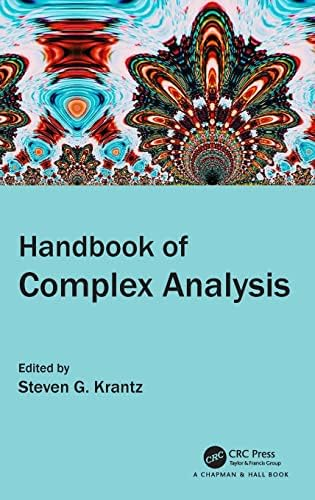Krantz, Steven G. (Ed.)
Handbook of complex analysis
Boca Raton, Florida : CRC Press, 2022. xi, 534 p.
QA300 H3523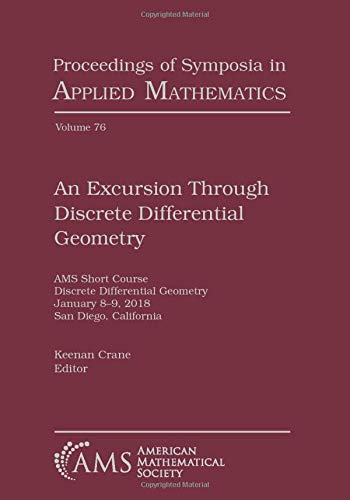Crane, Keenan
An excursion through discrete differential geometry
Providence, Rhode Island : American Mathematical Society, 2020. x, 140 p. -- (Proceedings of symposia in applied mathematics ; vol. 76)
QA649 A54 2020Magal, Pierre
Theory and applications of abstract semilinear Cauchy problems
Cham, Switzerland : Springer, 2018. xxii, 543 p.  -- (Applied mathematical sciences ; vol. 201)
QA377 M34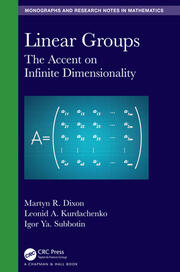Dixon, Martyn Russell
Linear groups : the accent on infinite dimensionality
Boca Raton, Florida : CRC Press, 2020. xiii, 314 p. -- (Monographs and research notes in mathematics)
QA252.3 D59Dobrev, Vladimir (Ed.)
Quantum theory and symmetries with Lie theory and its applications in physics
Singapore : Springer, 2018. xv, 427 p. (Springer proceedings in mathematics & statistics ; vol. 263)
QC173.96 I567 2017 v. IParanjape,  M. B. (Ed.)
Quantum theory and symmetries
Cham, Switzerland : Springer, 2021. xvii, 670 p. --  (CRM series in mathematical physics)
QC173.96 I567 2019Rocha-Arteaga, Alfonso
Topics in infinitely divisible distributions and Lévy processes
Cham, Switzerland : Springer, 2019. viii, 135 p. -- (SpringerBriefs in probability and mathematical statistics)
QA274.73 R63 2019Emmer, Michele (Ed.)
Mathematics and culture. Vol. 1
Berlin : Springer Verlag, 2004. viii, 352 p. -- (Mathematics and culture collection)
QA1 M38713 v. IEmmer, Michele (Ed.)
Mathematics and culture. Visual perfection: mathematics and creativity. Vol. 2.
Berlin : Springer Verlag, 2005. x, 203 p. -- (Mathematics and culture collection)
QA1 M38713 v. IIEmmer, Michele (Ed.)
Mathematics and culture. Vol. 3
Berlin : Springer Verlag, 2012. viii, 280 p. -- (Mathematics and culture collection)
QA1 M38713 v. IIIEmmer, Michele (Ed.)
Mathematics and culture. Vol. 5
Berlin : Springer Verlag, 2007. x, 269 p. -- (Mathematics and culture collection)
QA1 M38713 v. VZhang, Jun
Quantitative Tamarkin theory
Cham, Switzerland : Springer : 2020. x, 146 p. -- (CRM short courses)
QA665 Z43

### ¿Quién está en línea?

Hay 185 invitados y un miembro en línea

Go to top